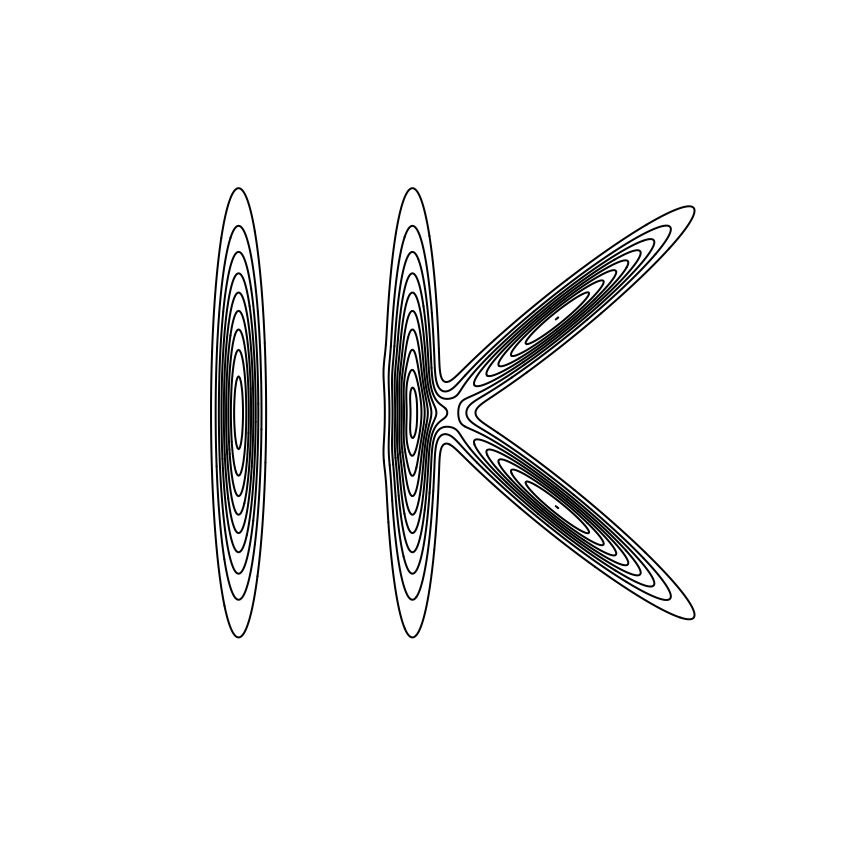My logo in GitHub (see here) is the contours of the density function of a mixture of bivariate normal distributions (see Figure 1). The idea came to me when I was working on the paper

• Kosmidis I and Karlis D (2016). Model-based clustering using copulas with applications.
Statistics and Computing, 26, 1079–1099
DOI ArXiV

In that paper, amongst several other things, we introduce the use of rotations of the components of mixtures of copulas to define extremely flexible mixture models. These models are fitted using the Expectation/Conditional Maximization (ECM) algorithm of Meng and Rubin (1993).

Back to the logo… The following chunk of R code is what generates it.

library("mvtnorm")
rotmat <- function(theta) {
co <- cos(theta)
si <- sin(theta)
matrix(c(co, si, -si, co), 2, 2)
}
## Create the grid
N <- 200
x <- seq(-6, 6, length = N)
x_grid <- expand.grid(x, x)
## Evaluate the density
sigma1 <- matrix(c(0.05, 0, 0, 5), 2, 2)
sigma2 <- matrix(c(0.05, 0, 0, 2.5), 2, 2)
d1 <- matrix(dmvnorm(x_grid, c(-4, 0), sigma1), N, N)
d2 <- matrix(dmvnorm(x_grid, c(-1, 0), sigma1), N, N)
d3 <- matrix(dmvnorm(x_grid, c(1.5, -2), rotmat(pi/4) %*% sigma2 %*% t(rotmat(pi/4))), N, N)
d4 <- matrix(dmvnorm(x_grid, c(1.5, 2), rotmat(-pi/4) %*% sigma2 %*% t(rotmat(-pi/4))), N, N)
d <- 0.3 * d1 + 0.3 * d2 + 0.4 * (d3 + d4)/2
contour(x, x, d, 12, drawlabels = FALSE, bty = "n", xaxt = "n", yaxt = "n")Figure 1: My logo